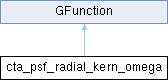GammaLib  1.7.0.dev

Kernel for radial model azimuth angle integration. More...

#include <GCTAResponse_helpers.hpp>## Public Member Functions

cta_psf_radial_kern_omega (const GCTAResponseCube *rsp, const GModelSpatialRadial *model, const GSkyDir &srcDir, const GEnergy &srcEng, const GTime &srcTime, const double &cos_psf, const double &sin_psf)

double eval (const double &omega)
Kernel for radial model integration over azimuth angle. More...Public Member Functions inherited from GFunction
GFunction (void)
Void constructor. More...

GFunction (const GFunction &function)
Copy constructor. More...

virtual ~GFunction (void)
Destructor. More...

GFunctionoperator= (const GFunction &function)
Assignment operator. More...

## Public Attributes

const GCTAResponseCubem_rsp
CTA response. More...

GSkyDir m_srcDir
True photon sky direction. More...

GEnergy m_srcEng
True photon energy. More...

GTime m_srcTime
True photon time. More...

double m_cos_psf
Cosine term for PSF offset angle computation. More...

double m_sin_psf
Sine term for PSF offset angle computation. More...Protected Member Functions inherited from GFunction
void init_members (void)
Initialise class members. More...

void copy_members (const GFunction &function)
Copy class members. More...

void free_members (void)
Delete class members. More...

## Detailed Description

Kernel for radial model azimuth angle integration.

This class implements the computation of

$K(\omega | \rho, E, t) = PSF(\omega | \rho)$

where $$PSF(\omega | \rho)$$ is the point spread function, $$\rho$$ is the distance from the model centre, and $$\omega$$ is the position angle with respect to the connecting line between the model centre and the observed photon arrival direction.

Definition at line 1103 of file GCTAResponse_helpers.hpp.

## Constructor & Destructor Documentation

 cta_psf_radial_kern_omega::cta_psf_radial_kern_omega ( const GCTAResponseCube * rsp, const GModelSpatialRadial * model, const GSkyDir & srcDir, const GEnergy & srcEng, const GTime & srcTime, const double & cos_psf, const double & sin_psf )
inline

Definition at line 1105 of file GCTAResponse_helpers.hpp.

## Member Function Documentation

 double cta_psf_radial_kern_omega::eval ( const double & omega )
virtual

Kernel for radial model integration over azimuth angle.

Parameters

Computes

$K(\omega | \rho, E, t) = PSF(\omega | \rho)$

where $$\omega$$ is the azimuth angle with respect to the model centre, counted counterclockwise from the vector connecting the model centre to the observed photon direction, and $$\rho$$ is the radial distance from the model centre.

From the coordinates $$(\rho,\omega)$$ in the model system, the method computes the angle between the true ( $$\vec{p}$$) and observed ( $$\vec{p'}$$) photon arrival direction using

$\delta = \arccos(\cos \rho \cos \rho_{\rm obs} + \sin \rho \sin \rho_{\rm obs} \cos \omega)$

where $$\rho_{\rm obs}$$ is the angular distance between the observed photon arrival direction $$\vec{p'}$$ and the model centre $$\vec{m}$$. $$\delta$$ is used to compute the value of the point spread function.

Implements GFunction.

Definition at line 1770 of file GCTAResponse_helpers.cpp.

## Member Data Documentation

Cosine term for PSF offset angle computation.

Definition at line 1126 of file GCTAResponse_helpers.hpp.

Referenced by eval().

Definition at line 1122 of file GCTAResponse_helpers.hpp.

CTA response.

Definition at line 1121 of file GCTAResponse_helpers.hpp.

Referenced by eval().

Sine term for PSF offset angle computation.

Definition at line 1127 of file GCTAResponse_helpers.hpp.

Referenced by eval().

True photon sky direction.

Definition at line 1123 of file GCTAResponse_helpers.hpp.

Referenced by eval().

True photon energy.

Definition at line 1124 of file GCTAResponse_helpers.hpp.

Referenced by eval().